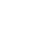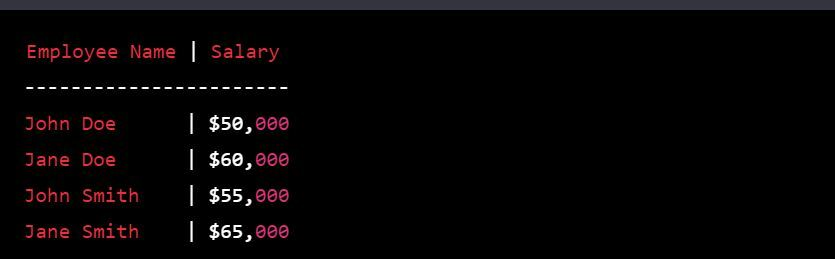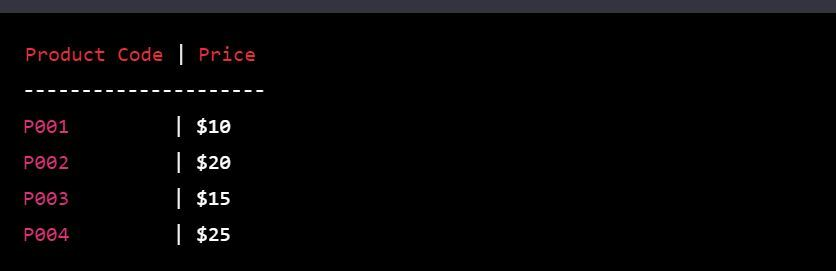Back to the list

# How to use a VLOOKUP in Microsoft Excel

## Formula: VLOOKUP

Is a function in Microsoft Excel that allows you to search for a value in the first column of a table and return a corresponding value from another column in the same row. It's commonly used to combine information from multiple sources, such as looking up an employee's name to find their salary, or finding the price of a product based on its code. The syntax for VLOOKUP is as follows:VLOOKUP(lookup_value, table_array, col_index_num, [range_lookup])

• lookup_value is the value you want to search for in the first column of the table.
• table_array is the range of cells that make up the table, including the first column where the lookup_value is searched for.
• col_index_num is the number of the column in the table_array that contains the value you want to return.
• [range_lookup] is an optional argument that specifies whether to find an exact or an approximate match. If it's set to TRUE (or omitted), an approximate match is returned. If it's set to FALSE, an exact match is required.

Here's an example of how to use VLOOKUP in Microsoft Excel:

Imagine you have a worksheet with a list of employees and their salaries, as follows:To find Jane Doe's salary, you can use the following formula:This formula will search for "Jane Doe" in the first column of the table (A2:B5), and return the value from the second column (col_index_num = 2) in the same row. The range_lookup argument is set to TRUE, so an approximate match is returned. The result will be \$60,000

Another example is to find the price of a product based on its code. Let's say you have a table with product codes and prices, as follows:To find the price of product P003, you can use the following formula:This formula will search for "P003" in the first column of the table (A2:B5), and return the value from the second column (col_index_num = 2) in the same row. The result will be \$15.